# Counterbalance Valve (IL)

High-pressure regulation valve in an isothermal liquid system

Since R2020a

•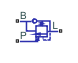Libraries:
Simscape / Fluids / Isothermal Liquid / Valves & Orifices / Pressure Control Valves

## Description

The Counterbalance Valve (IL) block represents a counterbalance pressure control valve in an isothermal liquid network. A counterbalance valve is common in applications where high-pressure events are common or when a task requires high-pressure controlled manipulation at production speeds This includes tasks such as overloading hydraulics or lowering suspended loads. The valve functions under the force balance between a spring, the back pressure at port B, and the load pressure at port L. The valve responds to changes in the pilot line pressure. When the pilot pressure exceeds the back pressure, the valve begins to open.

There is no flow between ports B and P or ports L and P.

This figure shows a typical counterbalance valve cutaway.### Valve Opening

The block balances the forces of the counterbalance valve, such that

`${p}_{pilot}{A}_{pilot}+{p}_{load}{A}_{load}={p}_{back}{A}_{back}+{F}_{set}+{k}_{spring}{x}_{spool},$`

where:

• ppilot is the pressure at port P.

• pload is the pressure at port L.

• pback is the pressure at port B.

• Fset is the accumulated force due to the spring.

• kspring is the spring constant.

• xspool is the linear position of the relief stage spool body. This position is relative to the unpressurized installed position.

The block sets the pressure-acting areas Apilot, Aload, and Aback according to the Pilot ratio parameter, Rpilot, such that

`${R}_{pilot}=\frac{{A}_{pilot}}{{A}_{load}},$`

and the Back pressure ratio parameter, Rback, such that

`${R}_{back}=\frac{{A}_{back}}{{A}_{load}}.$`

A ratio of 4:1 or 3:1 is typical for counterbalance valves.

The preset force, Fspring, represents the combined spring preloading and spring force at port B. This force is a function of pset, the Set pressure differential parameter, such that

`${F}_{set}={p}_{set}{A}_{load}.$`

This figure shows the schematic from the Counterbalance Valve With Test Harness example where the pressure ports control the valve by acting on surfaces with differential area. The arrows show the direction of force due to pressure or spring force acting on the relief stage body. The gray sections represent a single, solid body that moves to the right when the relief stage opens. The check valve travels to the left when it opens.### Opening Parameterization

The block accepts linear or tabulated parameterizations. The check valve portion of the block operates identically to the Check Valve (IL) block.

Linear Parameterization

When you set Opening parameterization to `Linear - Area vs. pressure`, the block calculates the valve opening area, such that

`${A}_{valve}=\stackrel{^}{p}\left({A}_{max}-{A}_{leak}\right)+{A}_{leak}.$`

The block calculates the normalized control pressure, $\stackrel{^}{p}$, as

`$\stackrel{^}{p}=\frac{\left({p}_{load}+{p}_{pilot}{R}_{pilot}-{p}_{back}{R}_{back}\right)-{p}_{set}}{\left({p}_{\mathrm{max}}-{p}_{set}\right)},$`

where pmax is the Maximum opening pressure differential parameter.

The normalized check valve pressure is

`$\stackrel{^}{p}=\frac{{p}_{control}-{p}_{cracking}}{{p}_{max}-{p}_{cracking}},$`

where:

• pcracking is the Cracking pressure differential parameter.

• pmax is the check valve Maximum opening pressure differential parameter.

When the valve is in a near-open or near-closed position in the linear parameterization, you can maintain numerical robustness in your simulation by adjusting the parameter. If the parameter is nonzero, the block smoothly saturates the control pressure between pcracking and pmax. For more information, see Numerical Smoothing.

Tabulated Area Parameterization

When you set Opening parameterization to ```Tabulated data - Area vs. pressure```, the block uses the same equations as for the linear parameterization but with tabulated data. The tabulated data represents area with respect to a given control pressure, where Pcontrol = pload + pPilotRPilot - pBackRBack. The first element of the Pressure differential vector parameter is the set pressure, which is the control pressure when the relief stage begins to open. The last element defines the control pressure when the valve is fully open.

Tabulated Flow Rate Parameterization

When you set Opening parameterization to ```Tabulated data - Volumetric flow rate vs. pressure```, the volumetric flow rate equations refer to these quantities:

• ${\overline{\rho }}_{valve}$ is the simulated average density of relief stage flow.

• K is the flow coefficient through the relief stage.

• Δpvalve is the pressure drop across the relief stage during flow.

• Δpcrit is the critical pressure drop across the relief stage during flow.

• pcontrol is the simulated control pressure.

• pcontrol,TLU,Ref is an internally derived lookup table of changing control pressures.

• pset is the set pressure differential.

• Recrit is the Critical Reynolds number parameter.

• Cd is the Discharge coefficient parameter.

• ν is the kinematic viscosity.

• KTLU,Ref is an internally derived lookup table of changing flow coefficients as a function of valve area.

• ${\stackrel{˙}{V}}_{TLU,Ref}$ is the reference volumetric flow rate vector.

• ppilot,Ref, pback,Ref, pload,Ref are the pilot pressure, back pressure, and load pressure for the user-provided Reference volumetric flow rate vector parameter, respectively.

The block calculates the mass flow rate for the relief stage such that

`${\stackrel{˙}{m}}_{valve}={\overline{\rho }}_{valve}K\frac{\Delta {p}_{valve}}{{\left(\Delta {p}_{valve}^{2}+\Delta {p}_{crit}^{2}\right)}^{1/4}},$`

where:

Liquid flows from the load port to the back port. The block extrapolates K to the nearest point after it calculates KTLU,Ref as

`${K}_{TLU,Ref}=\frac{{\stackrel{˙}{V}}_{TLU,Ref}}{\sqrt{\Delta {p}_{valve,TLU,ref}}},$`

where Δpvalve,TLU,ref = pload,Ref - pback,Ref. The block calculates the control pressure as

`${p}_{control}={R}_{pilot}{p}_{pilot}+{p}_{load}-{R}_{back}{p}_{back}$`

and the reference control vector as

`${p}_{control,TLU,Ref}={R}_{pilot}{p}_{pilot,Ref}+{p}_{load,Ref}-{R}_{back}{p}_{back,Ref}+{p}_{offset},$`

where:

The block internally derives Δpflow,Ref, which is the pressure drop for fluid flow in the specified reference curve. The block calculates poffset internally as

`${p}_{offset}={p}_{set}-{R}_{pilot}{p}_{pilot,Ref}-{p}_{load,Ref}\left(1\right)+{R}_{back}{p}_{back,Ref}.$`

The table shows how the block controls the relief and check stages opening areas depending the Opening area parameter setting.

Opening parameterizationRelief stageCheck stage
```Linear - Area vs. pressure```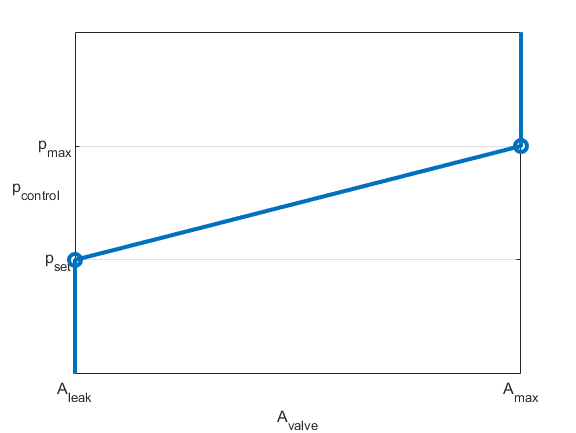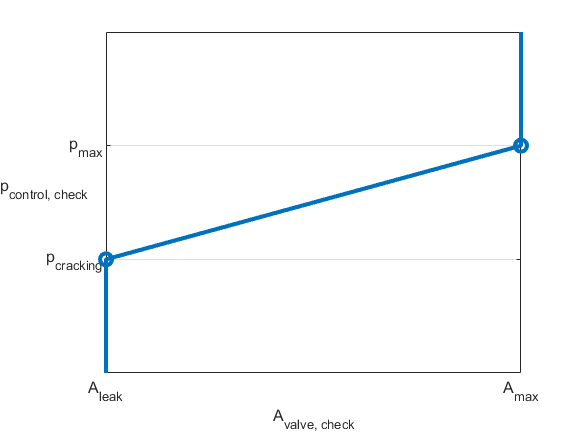```Tabulated - Area vs. pressure```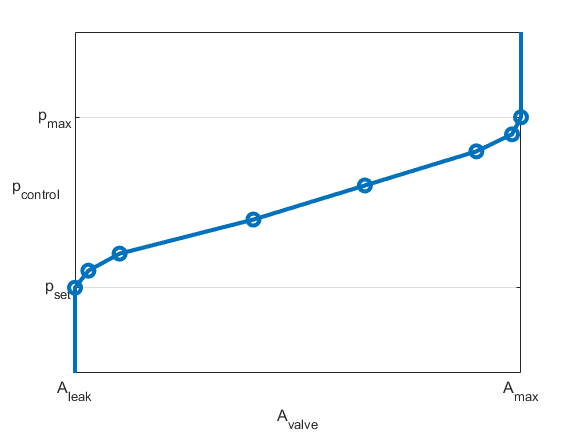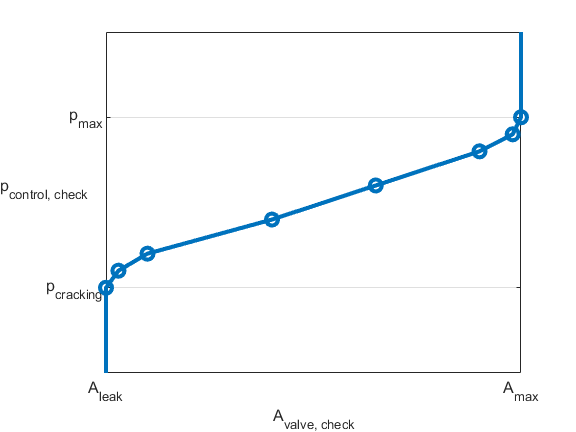```Tabulated - Volumetric flow rate vs. pressure```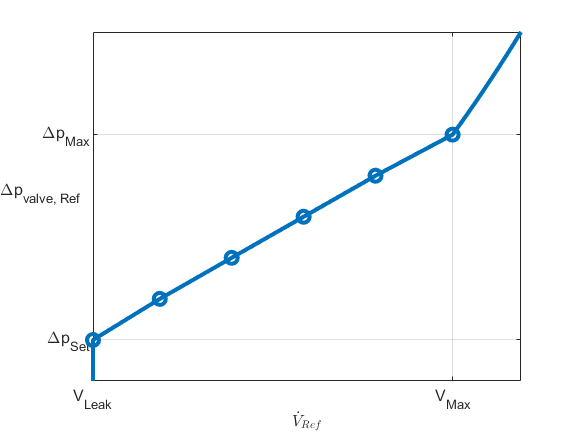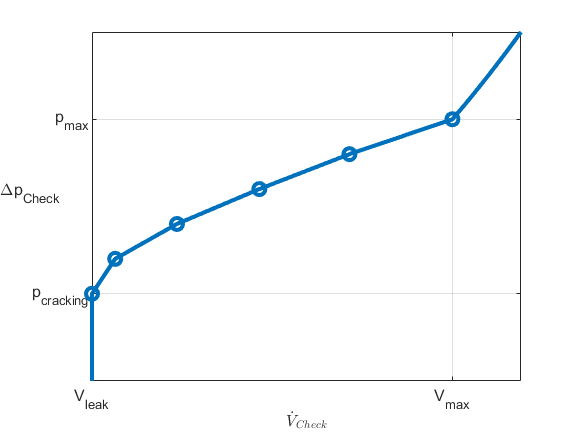### Predefined Parameterization

You can populate the block with pre-parameterized manufacturing data, which allows you to model a specific supplier component.

1. In the block dialog box, next to Selected part, click the "<click to select>" hyperlink next to Selected part in the block dialogue box settings.

2. The Block Parameterization Manager window opens. Select a part from the menu and click Apply all. You can narrow the choices using the Manufacturer drop down menu.

3. You can close the Block Parameterization Manager menu. The block now has the parameterization that you specified.

4. You can compare current parameter settings with a specific supplier component in the Block Parameterization Manager window by selecting a part and viewing the data in the Compare selected part with block section.

Note

Predefined block parameterizations use available data sources to supply parameter values. The block substitutes engineering judgement and simplifying assumptions for missing data. As a result, expect some deviation between simulated and actual physical behavior. To ensure accuracy, validate the simulated behavior against experimental data and refine your component models as necessary.

## Assumptions and Limitations

• The block operates in only the internal drain–external pilot, non-vented configuration.

• When you set Opening parameterization to ```Tabulated data - Volumetric flow rate vs. pressure```, the block uses values of volumetric flow rate with respect to the pressure drop across the relief stage to generate reference curves. The block assumes that the pilot port and back port are open to atmospheric pressure.

## Ports

### Conserving

expand all

Isothermal liquid conserving port associated with the load.

Isothermal liquid conserving port associated with the back pressure of the valve.

Pilot pressure port.

## Parameters

expand all

### Model Parameterization

Method of modeling the opening of the valve. When you select `Linear - Area vs. pressure` or `Tabulated data - Area vs. pressure`, the block relates the opening area to the control pressure. When you select ```Tabulated data - Volumetric flow rate vs. pressure```, the block takes a reference flow rate and pressure differential curve for the relief and check stages of the counterbalance valve, where the pressure at the back port and pilot port corresponds to 1 atm in the reference curve for the relief stage.

Pressure differential to lift the relief stage. Use this parameter to adjust the set pressure supplied by the data sheet.

#### Dependencies

To enable this parameter, set Valve parameterization to ```Linear - Area vs. pressure``` or ```Tabulated data - Volumetric flow rate vs. pressure```.

Vector of pressure differential values for the relief stage. The vector elements must correspond one-to-one with the elements in the Opening area vector parameter. You must enter the elements in ascending order. This vector defines the control pressures that govern valve opening and shutting. The first element in the vector defines the set pressure, which is the control pressure when the relief stage just begins to open. The last element defines the control pressure for which the valve is fully open.

#### Dependencies

To enable this parameter, set Valve parameterization to ```Tabulated data - Area vs. pressure```.

Vector of pressure drops across the relief stage. The vector elements must correspond one-to-one with the elements in the Reference volumetric flow rate vector parameter.

#### Dependencies

To enable this parameter, set Valve parameterization to ```Tabulated data - Volumetric flow rate vs. pressure```.

Vector of flow rates through the relief stage. The vector elements must correspond one-to-one with the elements in the Reference pressure differential vector parameter.

#### Dependencies

To enable this parameter, set Valve parameterization to ```Tabulated data - Volumetric flow rate vs. pressure```.

The maximum relief valve pressure.

#### Dependencies

To enable this parameter, set Valve parameterization to ```Linear - Area vs. pressure```.

Maximum open area of the relief stage orifice.

#### Dependencies

To enable this parameter, set Valve parameterization to ```Linear - Area vs. pressure```.

Sum of all gaps when the valve is in the fully closed position. Any area smaller than this value is saturated to the specified leakage area. This value contributes to numerical stability by maintaining continuity in the flow.

#### Dependencies

To enable this parameter, set Valve parameterization to ```Linear - Area vs. pressure```.

Ratio of the pressure-acting area of the pilot to the pressure-acting area of the load.

Ratio of the back pressure pressure-acting area to the load pressure-acting area.

Correction factor that accounts for discharge losses in theoretical flows.

Upper Reynolds number limit for laminar flow through the valve.

Continuous smoothing factor that introduces a layer of gradual change to the flow response when the valve is in near-open or near-shut positions. Set this value to a nonzero value less than one to increase the stability of your simulation in these regimes.

Vector of relief stage opening areas. The vector elements must correspond one-to-one with the elements in the Pressure differential vector parameter. List the elements in ascending order. All elements must be greater than 0.

#### Dependencies

To enable this parameter, set Valve parameterization to ```Tabulated data - Area vs. pressure```.

Whether to account for the transient effects to the fluid system due to opening the valve. Selecting Opening dynamics approximates the opening conditions by introducing a first-order lag in the pressure response. The Opening time constant parameter also impacts the modeled opening dynamics.

When enabled, these dynamics apply to the relief stage and not the check stage of the counterbalance valve.

Constant that captures the time required for the fluid to reach steady-state conditions when opening or closing the valve from one position to another. This parameter impacts the modeled opening dynamics.

#### Dependencies

To enable this parameter, select Opening dynamics.

### Check Valve

Pressure differential that lifts the check valve.

#### Dependencies

To enable this parameter, set Valve parameterization to ```Linear - Area vs. pressure```.

Maximum valve pressure. This parameter bounds the pressures at the valve exit to maintain physical values during simulation.

#### Dependencies

To enable this parameter, set Valve parameterization to ```Linear - Area vs. pressure```.

Maximum open area of the check valve in the load line. Internally, this check valve is in parallel with the relief stage.

#### Dependencies

To enable this parameter, set Valve parameterization to ```Linear - Area vs. pressure```.

Vector of pressure differentials for the check valve stage.

#### Dependencies

To enable this parameter, set Valve parameterization to ```Tabulated data - Area vs. pressure``` or ```Tabulated data - Volumetric flow rate vs. pressure```.

Vector of opening areas for the check valve stage. The vector elements must correspond one-to-one with the elements in the Pressure differential vector parameter.

#### Dependencies

To enable this parameter, set Valve parameterization to ```Tabulated data - Area vs. pressure```.

Vector of volumetric flow rates for the check valve stage. The elements of this vector must correspond to the elements in the Pressure differential vector parameter.

#### Dependencies

To enable this parameter, set Valve parameterization to ```Tabulated data - Volumetric flow rate vs. pressure```.

## Version History

Introduced in R2020a

expand all